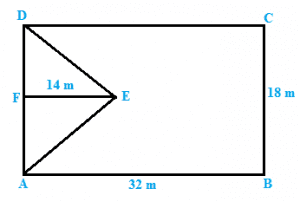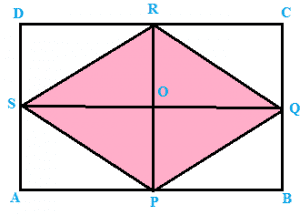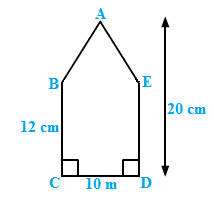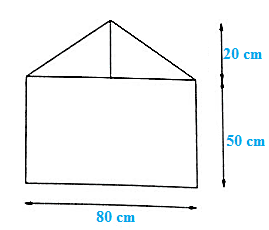×#### Thank you for registering.

One of our academic counsellors will contact you within 1 working day.

Click to Chat

1800-1023-196

+91-120-4616500

CART 0

• 0

MY CART (5)

Use Coupon: CART20 and get 20% off on all online Study Material

ITEM
DETAILS
MRP
DISCOUNT
FINAL PRICE
Total Price: Rs.

There are no items in this cart.
Continue Shopping• Complete JEE Main/Advanced Course and Test Series
• OFFERED PRICE: Rs. 15,900
• View Details

```Mensuration I (Perimeter and Area of Rectilinear Figures) Exercise 20.4

Question: 1

Find the area in square centimeters of a triangle whose base and altitude are as under :

(i) base =18 cm, altitude = 3.5 cm

(ii) base = 8 dm, altitude =15 cm

Solution:

We know that the area of a triangle = 1/2 (Base x Height)

(i) Here, base = 18 cm and height = 3.5 cm

Area of the triangle = 1/2 x 18 x 3.5

= 31.5 cm2

(ii) Here, base = 8 dm = (8 x 10) cm = 80 cm [Since 1 dm = 10 cm] and height = 3.5 cm

Area of the triangle = 1/2 x 80 x 15

= 600 c m2

Question: 2

Find the altitude of a triangle whose area is 42 cm2 and base is 12 cm.

Solution:

We have,

Attitude of a triangle = (2 x Area)/Base

Here, base = 12 cm and area = 42 cm2

Attitude = (2 x 42)/12 = 7 cm

Question: 3

The area of a triangle is 50 cm2. If the altitude is 8 cm, what is its base?

Solution:

We have,

Base of a triangle = (2 x Area)/ Altitude

Here, altitude = 8 cm and area = 50 cm2

Altitude = (2 x 50)/ 8 = 12.5 cm

Question: 4

Find the area of a right angled triangle whose sides containing the right angle are of lengths 20.8 m and 14.7 m.

Solution:

In a right-angled triangle,

The sides containing the right angles are of lengths 20.8 m and 14.7 m.

Let the base be 20.8 m and the height be 141 m.

Then,

Area of a triangle = 1/2 (Base x Height)

= 1/2 (20.8 × 14.7)

= 152.88 m2

Question: 5

The area of a triangle, whose base and the corresponding altitude are 15 cm and 7 cm, is equal to area of a right triangle whose one of the sides containing the right angle is 10.5 cm. Find the other side of this triangle.

Solution:

For the first triangle, we have,

Base = 15 cm and altitude = 7 cm

Thus, area of a triangle = 1/2 (Base x Altitude)

= 1/2 (15 x 7)

= 52.5 cm2

It is given that the area of the first triangle and the second triangle are equal.

Area of the second triangle = 52.5 c m2

One side of the second triangle = 10.5 cm

Therefore, The other side of the second triangle = (2 x Area)/One side of a triangle

= (2x 52.5)/10.5

=10 cm

Hence, the other side of the second triangle will be 10 cm.

Question: 6

A rectangular field is 48 m long and 20 m wide. How many right triangular flower beds, whose sides containing the right angle measure 12 m and 5 m can be laid in this field?

Solution:

We have,

Length of the rectangular field = 48 m

Breadth of the rectangular field = 20 m

Area of the rectangular field = Length x Breadth = 48 m x 20 m = 960 m2

Area of one right triangular flower bed = ½ (12 m x 5m) = 30 m2

Therefore,

Required number of right triangular flower beds = 960 m2/30 m2= 32

Question: 7

In Figure, ABCD is a quadrilateral in which diagonal AC = 84 cm; DL ⊥AC, BM ⊥ AC, DL = 16.5 cm and BM = 12 cm. Find the area of quadrilateral ABCD.Solution:

We have,

AC = 84 cm, DL = 16.5 cm and BM = 12 cm

Area of triangle ADC = 1/2 (AC x DL) = 1/2 (84 cm x 16.5 cm) = 693 cm2

Area of triangle ABC = 1/2 (AC x BM) = 1/2 (84 cm x 12 cm) = 504 cm2

Hence, Area of quadrilateral ABCD = Area of ADC + Area of ABC = (693 + 504) cm2 = 1197 cm2

Question: 8

Find the area of the quadrilateral ABCD given in Figure. The diagonals AC and BD measure 48 m and 32 m respectively and are perpendicular to each other.Solution:

We have,

Diagonal AC = 48 cm and diagonal BD = 32 m

Area of a quadrilateral = 1/2 (Product of diagonals)

= 1/2(AC x BD) = 1/2 (48 x 32) m2

= (24 x 32) m2 = 768 m2

Question: 9

In Fig below, ABCD is a rectangle with dimensions 32 m by 18 m. ADE is a triangle such that EF⊥ AD and EF= 14 cm. Calculate the area of the shaded region.Solution:

We have,

Area of the rectangle = AB x BC = 32 m x 18 m = 576 m2

Area of the triangle = 1/2 (AD x FE)

= 1/2 (BC x FE) [Since AD = BC]

= 1/2 (18 m x 14 m)

= 9 m x 14 m

= 126 m2

Area of the shaded region = Area of the rectangle – Area of the triangle

= (576 – 126) m2

= 450 m2

Question: 10

In Fig. below, ABCD is a rectangle of length AB = 40 cm and breadth BC = 25 cm. If P, Q, R, S be the mid-points of the sides AB, BC, CD and DA respectively, find the area of the shaded region.Solution:

Join points PR and SQ. These two lines bisect each other at point 0.Here, AB = DC = SQ = 40 cm and AD = BC = RP = 25 cm

Also OP = OR = RP/2 = 25/2 = 12.5 cm

From the figure we observed that,

Area of Triangle SPQ = Area of Triangle SRQ

Hence, area of the shaded region = 2 x (Area of SPQ)

= 2 x (1/2 (SQ x OP))

= 2 x (1/2 (40 cm x 12.5 cm))

= 500 cm2

Question: 11

Calculate the area of the quadrilateral ABCD as shown in Figure, given that BD = 42 cm, AC = 28 cm, OD = 12 cm and AC ⊥ BO.Solution:

We have,

BD = 42 cm, AC = 28 cm, OD= 12 cm

Area of Triangle ABC = 1/2 (AC x OB)

= 1/2 (AC x (BD – OD))

= 1/2 (28 cm x (42 cm – 12 cm))

= 1/2 (28 cm x 30 cm)

= 14 cm x 30 cm

= 420 cm2

Area of Triangle ADC = 1/2 (AC x OD) = 1/2 (28 cm x 12 cm)

= 14 cm x 12 cm

= 168 cm2

Hence, Area of the quadrilateral ABCD = Area of ABC + Area of ADC

= (420 + 168) cm2

= 588 cm2

Question: 12

Find the area of a figure formed by a square of side 8 cm and an isosceles triangle with base as one side of the square and perimeter as 18 cm.

Solution:Let x cm be one of the equal sides of an isosceles triangle.

Given that the perimeter of the isosceles triangle = 18 cm

Then, x + x + 8 =18

2x = (18 – 8) cm = 10 cm

x = 5 cm

Area of the figure formed = Area of the square + Area of the isosceles triangleQuestion: 13

Find the area of Figure, in the following ways: (i) Sum of the areas of three triangles (ii) Area of a rectangle — sum of the areas of five trianglesSolution:

We have,

(i) P is the midpoint of AD.

Thus AP = PD = 25 cm and AB = CD = 20 cm

From the figure, we observed that,

Area of Triangle APB = Area of Triangle PDC

Area of Triangle APB = 1/2 (AB x AP ) = 1/2 (20 cm x 25 cm) = 250 cm2

Area of Triangle PDC = Area of Triangle APB = 250 c m2

Area of Triangle RPQ = 1/2 (Base x Height) = 1/2 (25 cm x 10 cm) = 125 cm2

Hence, Sum of the three triangles = (250 + 250 + 125) cm2 = 625 cm2

(ii) Area of the rectangle ABCD = 50 cm x 20 cm = 1000 cm2

Thus, Area of the rectangle – Sum of the areas of three triangles

= (1000 – 625 ) cm2 = 375 cm2

Question: 14

Calculate the area of quadrilateral field ABCD as shown in Figure, by dividing it into a rectangle and a triangle.Solution:

We have,

Join CE , which intersect AD at point E.Here, AE = ED = BC = 25 m and EC = AB = 30 m

Area of the rectangle ABCE = AB x BC = 30 m x 25 m = 750 m2

Area of Triangle CED = 1/2 (EC x ED) = 1/2 ( 30 m x 25 m) = 375 m2

Hence, Area of the quadrilateral ABCD = (750 + 375) m2 = 1125 m2

Question: 15

Calculate the area of the pentagon ABCDE, where AB = AE and with dimensions as shown in Figure.Solution:

Join BE.Area of the rectangle BCDE = CD x DE

= 10 cm x 12 cm

= 120 c m2

Area of Triangle ABE = 1/2 (BE x height of the triangle)

= 1/2 (10 cm x (20 – 12) cm)

= 1/2 (10 cm x 8 cm)

= 40 cm2

Hence, Area of the pentagon ABCDE = (120 + 40) cm2 = 160 cm2

Question: 16

The base of a triangular field is three times its altitude. If the cost of cultivating the field at Rs 24.60 per hectare is Rs 332.10, find its base and height.

Solution:

Let altitude of the triangular field be h m

Then base of the triangular field is 3h m.

Area of the triangular field = 1/2 (h x 3h )=3h2/2 m2 —–(i)

The rate of cultivating the field is Rs 24.60 per hectare.

Therefore,

Area of the triangular field = 332.10 /24.60

= 13.5 hectare = 135000 m2 [Since 1 hectare = 10000 m2] —–(ii)

From equation (i) and (ii) we have,

3h2/2 = 135000 m2

3h2 = 135000 x 2 = 270000 m2

h2 = 270000/3 m2= 90000 m2 = (300 m)2

h = 300 m

Hence, Height of the triangular field = 300 m and base of the triangular field = 3 x 300 m = 900 m

Question: 17

A wall is 4.5 m long and 3 m high. It has two equal windows, each having form and dimensions as shown in Figure. below. Find the cost of painting the wall (leaving windows) at the rate of Rs 15 per m2.Solution:

We have,

Length of a wall = 4.5 m

Breadth of the wall = 3 m

Area of the wall = Length x Breadth

= 4.5 m x 3 m = 13.5 m2

From the figure we observed that,

Area of the window = Area of the rectangle + Area of the triangle

= (0.8 m x 0.5 m) + (12 x 0.8 m x 0.2 m) [Since 1 m = 100 cm]

= 0.4 m2 + 0.08 m2

= 0.48 m2

Area of two windows = 2 x 0.48 = 0.96 m2

Area of the remaining wall (leaving windows) = (13.5 – 0.96) m2

= 12.54 m2

Cost of painting the wall per m2 = Rs. 15

Hence, the cost of painting on the wall = Rs. (15 x 12.54)

= Rs. 188.1

(In the book, the answer is given for one window, but we have 2 windows.)
```### Course Features

• 728 Video Lectures
• Revision Notes
• Previous Year Papers
• Mind Map
• Study Planner
• NCERT Solutions
• Discussion Forum
• Test paper with Video Solution